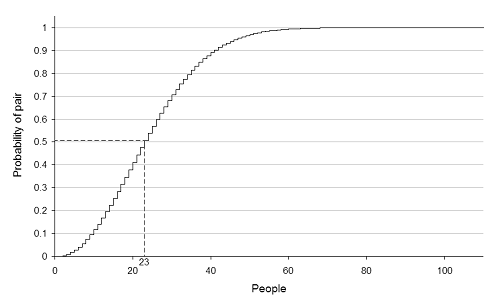A Life Spent Wondering

# The Birthday Problem

3,778 views Random

If someone came up to you in a party and said "I bet you that two people here have the same birthday," what would you say? It would seem rather unlikely given there are 365 days in a year. Maybe if there were hundreds of people, would you be more inclined to take the bet then?

It turns out that you don't need a giant group of people to have good odds in this situation. Using the power of math we can use probabilities to determine how many people need to be in a room for any two people to have 50% chance of sharing a birthday.

### It's sll in the math

If you flip a coin, you know that there is a 50% chance of it landing on heads and a 50% chance of it coming up tails. Let's say you call heads in the air. There is a 50% chance of you winning (success) and the other half, you lose (failure). As with any probability problem, the sum of the success and failure is equal to 1; in this case, 0.5 + 0.5 = 1.

We can use the same logic in the birthday problem by calling the chance of two people having the same birthday a success and the chance of two people not having the same birthday a failure. With two people, the chance of them not having the same birthday is:

(365/365) * (364/365) = .9972 = a 99.7% chance

365 days available for the first person

364 for the second person

Now let's consider the chance of 3 people not having the same birthday. This means that the first and second person do not have the same birthday and the third person does not have the same birthday as the first or second person.

(365/365) * (364/365) * (363/365) = .9918 = 98.2% chance

365 days available for the first person

364 for the second person

363 for the third person

With each person that is added on, the chance of all of them having a different birthday decreases.

The equation for n birthdays is:

365!

P(no bday in common) = ----------------------

(365-n)! * (365^n)

Keep in mind that the equation calculates the probability that n amount of people do not have the same birthday. This means that to calculate the probability of n people that do have the same birthday, we just subtract the probability of failure (no b-day matches) from 1 (the sum of the success and failure).

### The coin flip

So how many people need to be in a room for one person to have a 50% chance of sharing a birthday with another? By using the equation above, the variable n can be increased until we get a result around 50%. It turns out that for you to have a coin flip's chance, only n = 23 people need to be considered. In other words, in a room of 23 people, there is a 50% (technically 50.73%) chance that two of them will have the same birthday.

### Odds with over 23 people

Let's start increasing n further. At 30 people, there is a ~67% chance of two matching birthdays. At 40 people the probability increases to ~87%. Up at 57, the chance is better than 99%. The graph below illustrates this.image courtesy: wikipedia.org

So remember that if someone ever approaches you with "I bet you that two people here have the same birthday", if there are at least 23 people then it may be worth taking the bet!